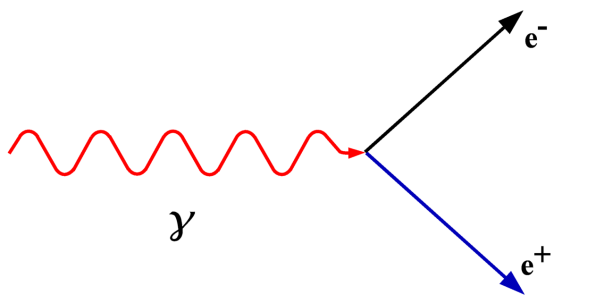# Why Some Particles Don’t DecayMost particles do not “live” forever. The neutron, a neutral particle which resides in the nucleus of every element except hydrogen, has an average lifetime of 882 seconds (just under quarter of an hour) when outside of the nucleus. The proton has a very long lifetime and has never been observed to decay, but as Murray Gell-Mann said “Everything not forbidden is compulsory”. We therefore know that because the proton can decay, it must (eventually) decay. (Current thinking is that the lower bound, the shortest possible half-life for the proton, is at least six billion trillion trillion years, much much longer than the age of the Universe.)

But some particles: the electron, the electron neutrino, and the photon do live forever. Why is this?

When a particle decays there are a number of quantities that must be conserved, i.e. they must be the same before and after the decay. Mass-energy is conserved, so the amount of mass and energy before and after the decay must be the same. Charge is conserved, so the total charge before and after the decay must be the same.

If we take the electron as an example, it is the lightest of the charged particles, with a mass of about one eighteen hundredth of the mass of a proton. When a particle decays it must decay into a lighter particle (otherwise where would the extra mass come from?) and it must obey the conservation rules explained above. But here we have a problem: there are particles lighter than the electron (e.g. the electron neutrino) and there are particles with the same charge as the electron (e.g. the muon), but there are no particles that are both lighter than the electron and which are charged. Because the decay of an electron would violate conservation laws it is forbidden, there is nothing it could decay into, and therefore decay does not occur.